Search
Document Center

# Implement image classification by TensorFlow

Last Updated: Oct 29, 2018

## Overview

The development of the Internet has generated large volumes of images and voice data. How to effectively make use of this unstructured data has always been a challenge for data mining professionals. The processing of unstructured data usually involves the use of deep learning algorithms. These algorithms can be daunting to use at first sight. In addition, processing this data usually requires powerful GPUs and a large amount of computing resources. This document introduces a method of image recognition using deep learning frameworks. This method can be applied to scenarios such as illicit image filtering, facial recognition, and object detection.

This guide creates an image recognition model using the deep learning framework TensorFlow in Alibaba Cloud Machine Learning Platform for AI. The entire procedure takes about 30 minutes to complete. After the procedure, the system is able to recognize the bird in the following image.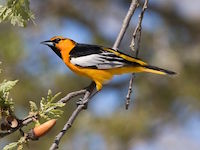## Dataset

The CIFAR-10 dataset is used in this guide. This dataset contains 60,000 32x32 color images in 10 different categories, such as airplanes, cars, birds, cats, deer, dogs, frogs, horses, ships, and trucks. The dataset is as follows.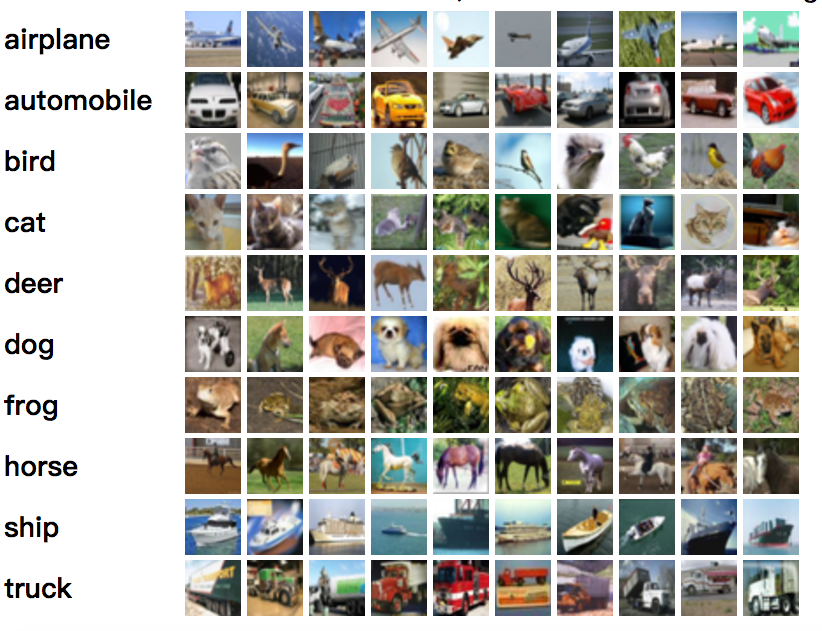This source data is divided into two parts: 50,000 images are used for training and 10,000 for testing. The 50,000 training images are further divided into five data_batch files, and the 10,000 testing images form a test_batch file. The source data contains the following.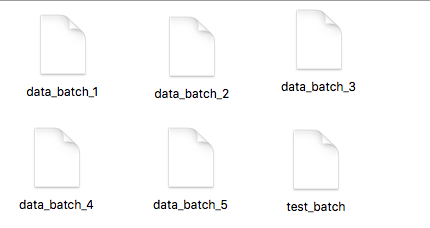## Training procedure

To create an experiment in the machine learning platform, you need to enable GPU usage and activate Object Storage Service (OSS) to store your data.

#### 1. Data preparation

2. Log on to OSS, and create an OSS bucket ( For more information, see OSS Document ).

3. Create new directory in OSS bucket. An aohai_test directory is created in this article, and four folders are created under this directory as follows.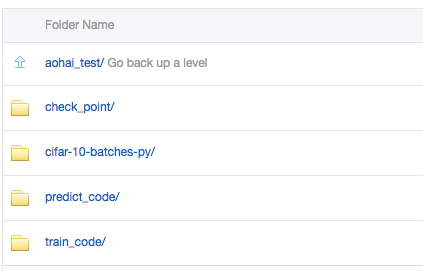The role of each folder is as follows:

• check_point: Stores the models that are generated in the experiment.

• cifar-10-batches-py: Stores the training data, file cifar-10-batcher-py. The prediction data, file bird_mount_bluebird.jpg.

• predict_code: Stores the code file cifar_predict_pai.py.

• train_code: Stores the code file cifar_pai.py.

4. Upload the dataset and source code to the corresponding directory of the OSS bucket.

#### 2. OSS permissions Configuration

Log on to the machine learning platform, and click Settings to configure OSS permissions, as shown in the following figure. For more information, see the “Read OSS buckets” chapter of Deep learning.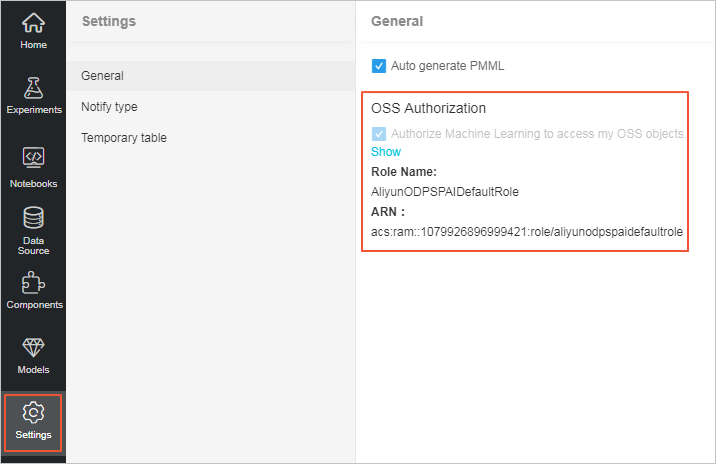#### 3. Model training

1. Drag a Read OSS Bucket component and a TensorFlow component to the canvas, and configure the TensorFlow component as follows.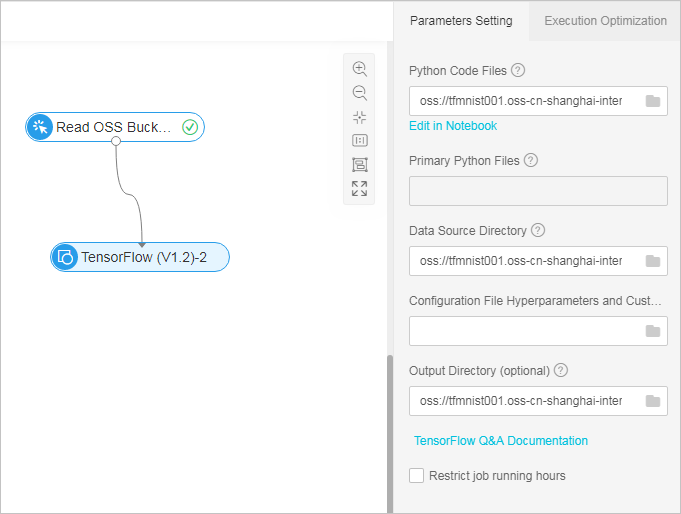• Python Code File: Select the OSS directory of cifar_pai.py.
• Data Source Directory: Select the OSS directory of cifar-10-batches-py.
• Output Directory: Select the OSS directory of check_point.
2. Click Run to start the training procedure.

You can change the number of GPUs by changing the configuration as follows. You can also adjust the number of GPUs in the code.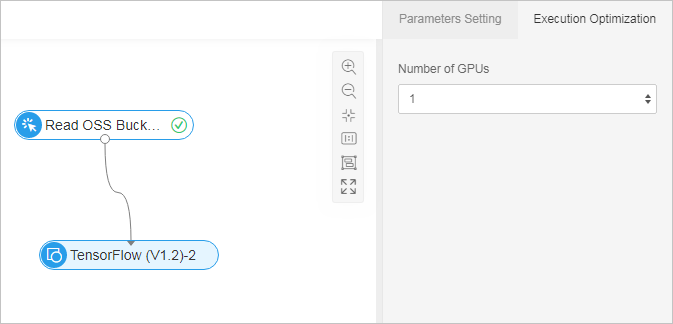#### 4. Training code explanation

Note the following code in cifar_pai.py:

• The following code creates the training model using the convolutional neural network (CNN).
``    network = input_data(shape=[None, 32, 32, 3],``                         data_preprocessing=img_prep,``                         data_augmentation=img_aug)``    network = conv_2d(network, 32, 3, activation='relu')``    network = max_pool_2d(network, 2)``    network = conv_2d(network, 64, 3, activation='relu')``    network = conv_2d(network, 64, 3, activation='relu')``    network = max_pool_2d(network, 2)``    network = fully_connected(network, 512, activation='relu')``    network = dropout(network, 0.5)``    network = fully_connected(network, 10, activation='softmax')``    network = regression(network, optimizer='adam',``                         loss='categorical_crossentropy',``                         learning_rate=0.001)``
• The following code generates the model model.tfl.
``    model = tflearn.DNN(network, tensorboard_verbose=0)``    model.fit(X, Y, n_epoch=100, shuffle=True, validation_set=(X_test, Y_test),``              show_metric=True, batch_size=96, run_id='cifar10_cnn')``    model_path = os.path.join(FLAGS.checkpointDir, "model.tfl")``    print(model_path)``    model.save(model_path)``

#### 5. Log view

1. Right-click the TensorFlow component to view the logs generated during the training process.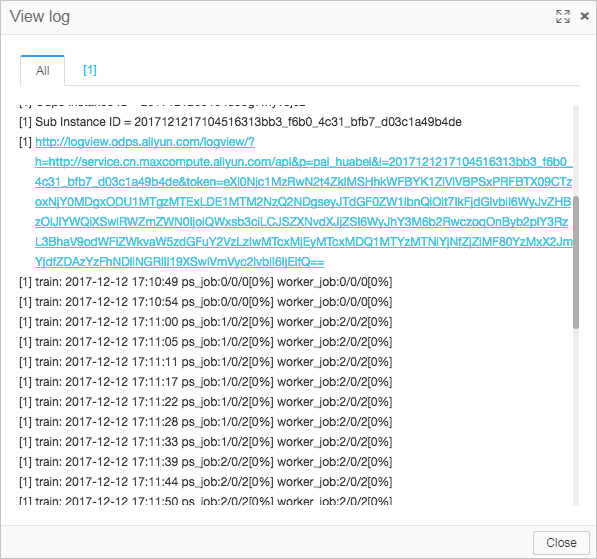2. Click a logview link and run the following steps to view the logs.

3. Click MWorker on the left, and choose All.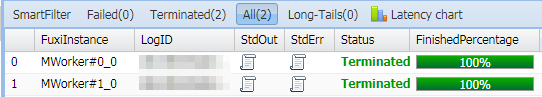4. Click StdOut to print the training logs.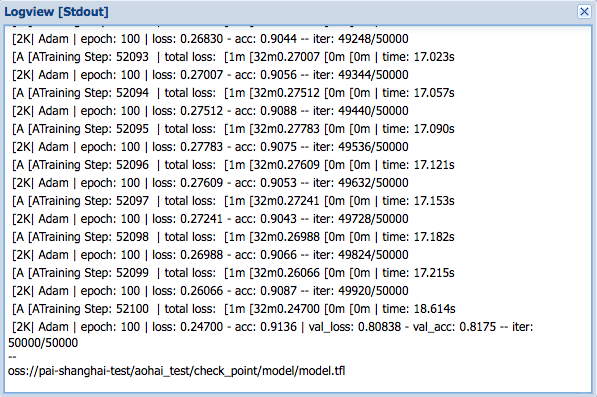More logs are printed as the experiment continues. You can also use the print function to print key information in the code. In this example, you can use the aac parameter to view the accuracy of the model.

#### 6. Result prediction

You can drag another TensorFlow component for use in predicting.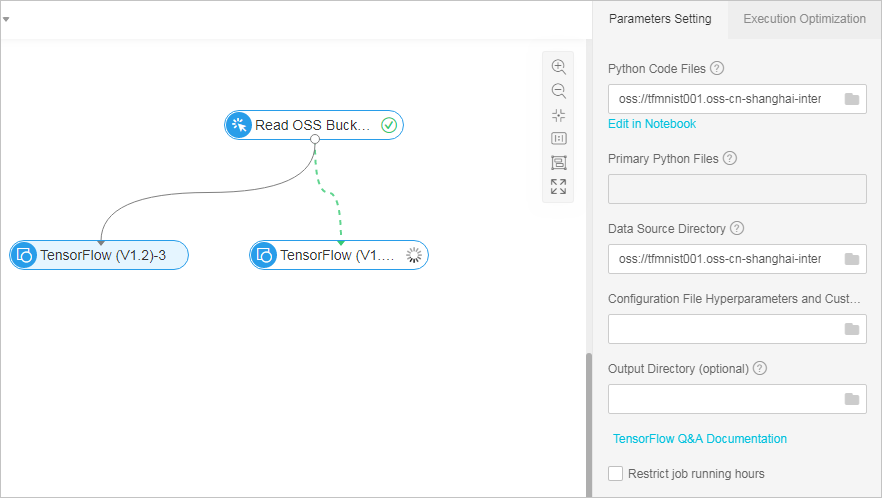• Python Code File: Select the OSS directory of cifar_predict_pai.py.
• Data Source Directory: Select the OSS directory of cifar-10-batches-py.
• Output Directory: Select the OSS directory of model model.tfl.

The image that is used for predicting is stored in the checkpoint folder.The prediction result is as follows: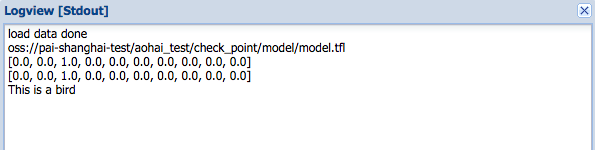#### 7. Predicting code explanation

The following code:

``  predict_pic = os.path.join(FLAGS.buckets, "bird_bullocks_oriole.jpg")``    img_obj = file_io.read_file_to_string(predict_pic)``    file_io.write_string_to_file("bird_bullocks_oriole.jpg", img_obj)``    img = scipy.ndimage.imread("bird_bullocks_oriole.jpg", mode="RGB")``    # Scale it to 32x32``    img = scipy.misc.imresize(img, (32, 32), interp="bicubic").astype(np.float32, casting='unsafe')``    # Predict``    prediction = model.predict([img])``    print (prediction)``    print (prediction)``    #print (prediction.index(max(prediction)))``    num=['airplane','automobile','bird','cat','deer','dog','frog','horse','ship','truck']``    print ("This is a %s"%(num[prediction.index(max(prediction))]))``
• Reads the image “bird_bullocks_oriole.jpg”, and scales the image to 32*32 pixels.
• Passes the image to the function `model.predict` to evaluate similarity scores.
• Returns the result based on the similarity scores. The class that scores the highest similarity is returned.

Note: Because of the randomness of the model training, it is not guaranteed that the model from each training can return accurate results for the predicted image. It is necessary to continuously debug the corresponding parameters to achieve a stable effect. This case is relatively simple and is for reference only.